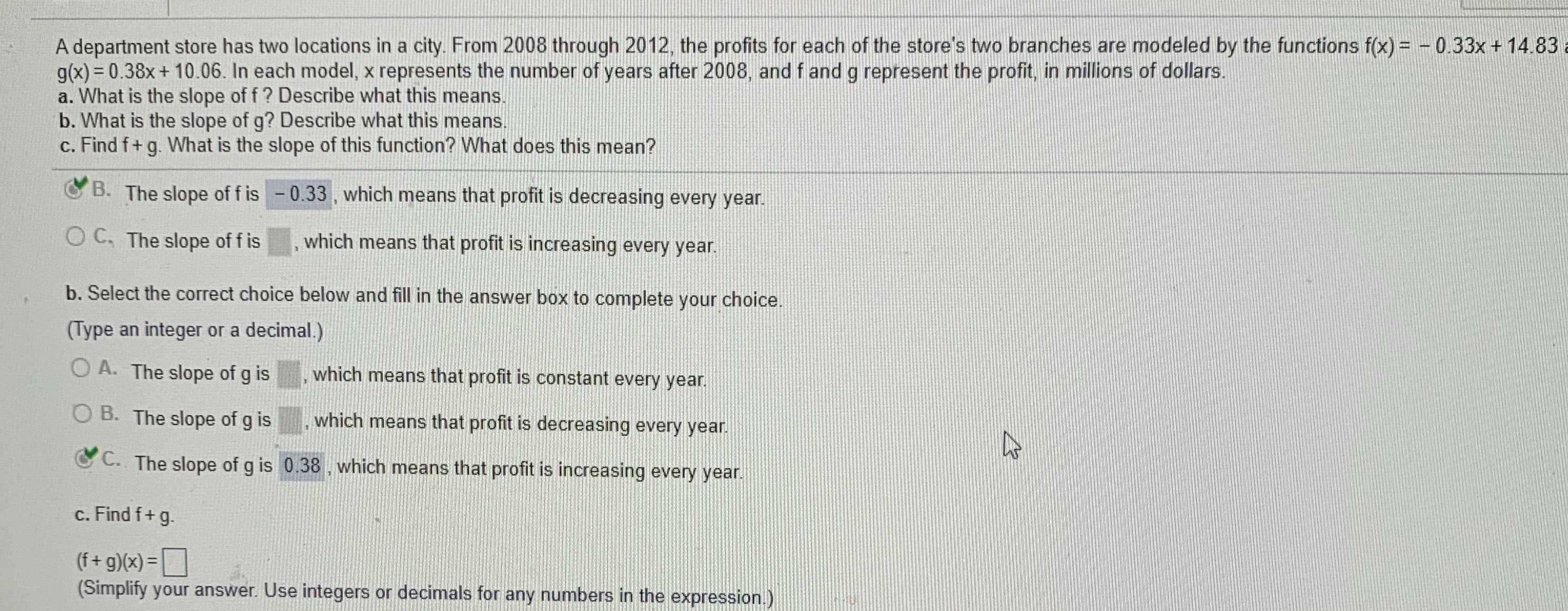### ¿Todavía tienes preguntas de matemáticas?

Pregunte a nuestros tutores expertos
Algebra
PreguntaA department store has two locations in a city. From $$2008$$ through $$2012$$ , the profits for each of the store's two branches are modeled by the functions $$f ( x ) = - 0.33 x + 14.83$$

$$g ( x ) = 0.38 x + 10.06$$ . In each model, $$x$$ represents the number of years after $$2008$$ , and $$f$$ and $$g$$ represent the profit, in millions of dollars. a. What is the slope of $$f$$ ? Describe what this means. b. What is the slope of g? Describe what this means. c. Find $$f + g$$ . What is the slope of this function? What does this mean?